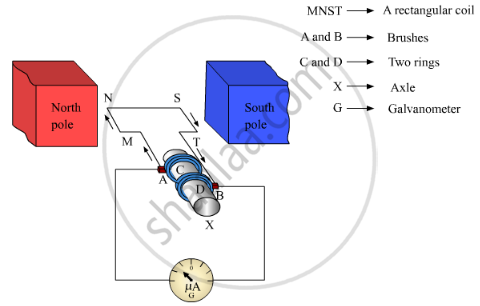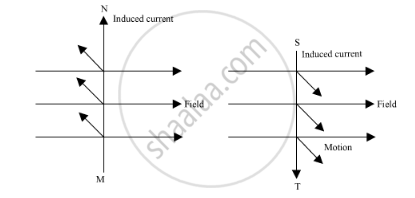# Explain the Construction and Working of the Following. Draw a Neat Diagram and Label It.Electric Generator (Ac) - Science and Technology 1

Explain the construction and working of the following. Draw a neat diagram and label it.

Electric Generator (AC)

#### Solution

Electric Generator principle : An electric generator is a machine that generates electricity by rotating its rotor in a magnetic field. Thus, it converts mechanical energy into electrical energy.

Construction: A generator consists of a rectangular coil MNST of insulated copper wire placed between two strong magnetic poles. The two ends of the coil MNST are connected with brushes A and B of rings C and D respectively. The inner sides of the rings are insulated. They are attached with an axle Xwhich can be rotated mechanically. Brushes A and B are connected with a galvanometer that can measure the flow of current in coil MNST.Working: When the axle is rotated, lengths MN and ST move up and down respectively. Since lengths MN and ST are moving in a magnetic field, a current gets induced in these lengths caused by an electromagnetic induction. The direction of the induced current in both the lengths is given by Fleming’s right hand rule.Since length MN is moving upwards in the magnetic field that acts from left to right, the direction of the induced current will be from M to N. Similarly, the direction of the induced current in length ST will be from S to T. Hence, an induced current will set up in the coil in the direction MNST, which produces deflection in the galvanometer.
After half-rotation, length MN starts moving down, whereas length ST starts moving up. The direction of the induced current in the coil gets reversed i.e., the induced current will now flow from T to M via S and N i.e., TSNM. Therefore, we can conclude that after each half-rotation, the direction of the induced current is reversed. This current is called an alternating current (AC). An AC reverses its direction after equal time intervals.

Concept: Alternating Current (A.C.) Generator
Is there an error in this question or solution?